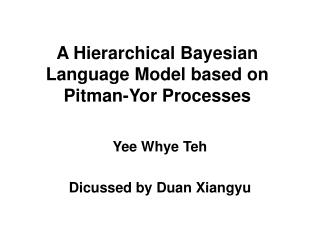Download PresentationA Hierarchical Bayesian Language Model based on Pitman-Yor Processes

A Hierarchical Bayesian Language Model based on Pitman-Yor Processes - PowerPoint PPT Presentation

A Hierarchical Bayesian Language Model based on Pitman-Yor Processes. Yee Whye Teh Dicussed by Duan Xiangyu. Introduction. N-gram language model This paper introduces hierarchical Baysian model for the above, that is, to modelI am the owner, or an agent authorized to act on behalf of the owner, of the copyrighted work described.
Download PresentationA Hierarchical Bayesian Language Model based on Pitman-Yor Processes

Download Policy: Content on the Website is provided to you AS IS for your information and personal use and may not be sold / licensed / shared on other websites without getting consent from its author.While downloading, if for some reason you are not able to download a presentation, the publisher may have deleted the file from their server.

- - - - - - - - - - - - - - - - - - - - - - - - - - E N D - - - - - - - - - - - - - - - - - - - - - - - - - -
Presentation Transcript
1. A Hierarchical Bayesian Language Model based on Pitman-Yor Processes Yee Whye Teh Dicussed by Duan Xiangyu

2. Introduction • N-gram language model • This paper introduces hierarchical Baysian model for the above, that is, to model • The hierarchical model in this paper is the hierarchical Pitman-Yor processes • Pitman-Yor processes can produce power-law distribution • Hierarchical structure is corresponding to smoothing techniques in language modeling.

3. Introduction of Pitman-Yor Processes • Let W be a vocabulary of V words, G(w) be the probability of a word w, and G=[G(w)]w∈W is the vector of word probabilities. • where base distribution G0=[G0(w)] w∈W, and G0(w)=1/V • d and θ are hyper-parameters.

4. Generative Procedure of PYP • A sequence of words: x1, x2,… drawn i.i.d from G • A sequence of draws y1, y2,… drawn i.i.d from G0 • With probability: , let xc.+1 = yk, that is, next word assigned to previous draw from G0 , letxc.+1 = yt+1, that is, next word assigned to new draw from G0 where t is the current number of draws from G0, ck is the number of words assigned to yk, and . This generative process of PYP exhibits rich get richer phenomenon

5. Metaphor to the Generative Procedure of PYP • Chinese Restaurant Process

6. Hierarchical PYP Language Models • Given context u, let Gu=[Gu(w)]w∈W • π(u) is the suffix of u consisting of all but the earliest word. For example, u is “1 2 3”, then π(u) is “2 3”. • Gπ(u)～PY(d|π(u)|, θ|π(u)|, Gπ(π(u))) • Until Gø～PY(d0, θ0, G0) This is hierarchy

7. Generative Procedure of Hierarchical PYP Language Models • Denotations: • xu1, xu2,… drawn from Gu • yu1, yu2,… drawn from Gπ(u) • We use l to index x, use k to index y. • tuwk=1 if yuk=w • cuwk is the number of words xul=yuk=w • We denote marginal counts by dots • cu.k is the number of words xul=yuk • cuw. is the number of words xul=w • tu.. is the number of draws yuk from Gπ(u)

8. cont.

9. Inference for Hierarchical PYP Language Models • We are interested in predictive probability: • We approximate it with {S(i),θ(i)}i=1I where